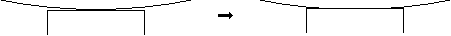+31(0)643 879 398 info(at)vinksda.nl
Select Page

The HertzWin program developed by Vink System Analysis & Design is used by many. Sometimes these users have questions in relation to the accuracy of the HertzWin results. Some of them are discussed here.

### Does the program contain computational errors (bugs)?

In addition to extensive in-house checks, various users have tested HertzWin and compared it to their own calculations. ASML, for example, subjected HertzWin to an extensive test program. All checks to date have not revealed any errors in the results. This, of course, does not guarantee that HertzWin is 100% bug-free. It is always wise to verify whether the results correspond to that what may be expected on the basis of a rough assessment.

### Proper application of the results

Although the HertzWin program is user-friendly, the results must be carefully examined. Mistakes with the HertzWin results are easily made. One of the more common mistakes to make is that the calculated contact radius of the point contact is not taken into account. For example:

Suppose there is a need to calculate the contact stresses of a roller with a curved surface, see the illustration below.Narrow roller with and without deformation.

This concerns an elliptic contact that can be easily calculated using HertzWin. However, bear in mind that the roller must be finite in width and that the contact width cannot exceed the width of the roller. If the calculated contact width is larger, there no longer is an elliptic contact and the formulas of Heinrich Hertz no longer apply. In that case, the results of HertzWin are inaccurate.

An other issue is that in line contacts, the ‘Hertz’-equations assume infinite length of the roller(s). In practice, the roller(s) is (are) of finite length and at the ends of the roller(s) higher stress can occur. Also the effects of misalignment, causing an unequal stress distribution over the contact area, are not taken into account.

### What is the level of accuracy of the formulas as derived by Heinrich Hertz?

The accuracy of the formulas by Heinrich Hertz has been subject to intensive research. For example, an article by Anantha Ram B.S. et all at the Aalborg University in Denmark, describes a study within the framework of the development of an abrasion model. The conclusion in this article is that “the results of the finite element analysis correspond well to the theoretic results. The difference between both is <2.5%.” Since the accuracy of the finite element analysis is usually around 1 – 2 %, it is safe to assume that the formulas of Heinrich Hertz produce exact results. The following preconditions must of course be met here:

• the contact surface of both objects is small compared to the radius of the objects;
• contact surfaces are perfectly smooth (no surface roughness);
• materials distort linearly elastically and are homogeneous;
• there is no friction.

Note: a widely used limit for the contact surface dimensions is 10% of the contacting radii. If the contact surface dimensions are within 20% of the contacting radii, the results of HertzWin are less accurate, but still acceptable in many cases.